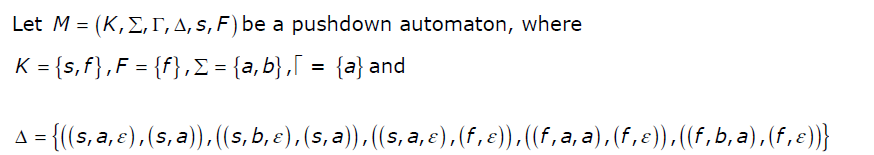# GATE | GATE-IT-2004 | Question 40

• Last Updated : 28 Jun, 2021

Which one of the following strings is not a member of L (M)?(A) aaa
(B) aabab
(C) baaba
(D) bab

Explanation:
Basics of PDA
A push down automata or a PDA is essentially a NFA with a stack and its transition function also depends on the symbol at the stack top. Formally, a PDA is a 6-tuple (Q, Σ, Γ, δ, q0, F) with Q being set of all possible states, Σ is the set of all possible input, Γ is the set of all possible stack symbol, δ : Q × Σ × Γ → Q × Γ is the transition function, q0 is the initial state, and
F ⊆ Q is the set of final state.

Attention reader! Don’t stop learning now.  Practice GATE exam well before the actual exam with the subject-wise and overall quizzes available in GATE Test Series Course.

Learn all GATE CS concepts with Free Live Classes on our youtube channel.

Some times, PDA is also known to be of 7 tuples when we considers the initial stack symbol z0 as an additional member in the
above 6 tuples. Note that there should exists a transition function for a given input otherwise there won’t be any possible move.

The PDA can be of two types: Empty stack accepting PDA and Final state PDA.

Empty stack accepting PDAs are the PDAs for which a given input is accepted if on a given input string, when the input exhausts, the stack should be empty.

Similarly, a Final state accepting PDA is a PDA for which a given input is accepted if on a given input string, when the input exhausts, thePDA should be in one of the final states.

A Non-deterministic PDA or NPDA is a PDA in which, for a given input, multiple output is possible. i.e. The Transition function δ maps a given input from Q × Σ × Γ to a finite set of Q × Γ.

For the given question
, since there is a more than one output corresponding to a given input for transition function, we will consider a NPDA for the execution of the input and we will halt our execution for a corresponding branch if there is no move possible for a given input and We assume that if the machine halts at one of the final state and input is exhausted, we accept the string otherwise we reject it. Notice, the Given PDA is final state accepting PDA and not the empty stack accepting PDA.

Choice of the Questions:

We will assume the initial stack symbol is ε, and

In choice 1, The execution will be as follow:
(s, a, ) → (s, a) or (f, ε).
Let’s first consider, (s, a) as output and execute the remaining input. (s,a, a) → No valid Move. halt this branch and not in the final state, so not accepted. Now consider (f, ε) as output. (f, a, ε) → No Valid Move.
halt this branch Notice that the PDA is in one of the final state, But the input string is not exhausted, So this should not be accepted by the PDA.

In choice 2, The Execution will start as of choice 1 and will halt at character 2 as no possible move. This option is also not accepted in same way as of the first option.
3

In choice 3, The execution will be as follow:
(s, b, ε) → (s, a). (s, a, a) → No Possible move. halt this branch. Since halted before reaching to any final state so this option is not accepted.

In choice 4, This option is also not accepted as the PDA will halt at second character as it halted in previous option. This option is also not accepted.

Assuming that if the PDA halts on a input due to no possible move and if the current state is one of the final state but the input is not exhausted, PDA will reject that string, For Question 2, Every answer is true i.e. None of the given for string belongs to the given PDA’s language.

Again, Assuming that if the PDA halts on a input due to no possible move and if the current state is one of the final state but the input is not exhausted, the PDA will keep on consuming the input string until the end of string comes, string in option (A) and option (B) will be accepted by the language of this PDA. while option (C) and option (D) still won’t get accepted as by the end
of the string, the PDA won’t be in final state. So keeping this assumption in mind, option (C) and option (D) both will be true for this question.

This explanation is contributed by Durgesh Pandey.

Quiz of this Question

My Personal Notes arrow_drop_up